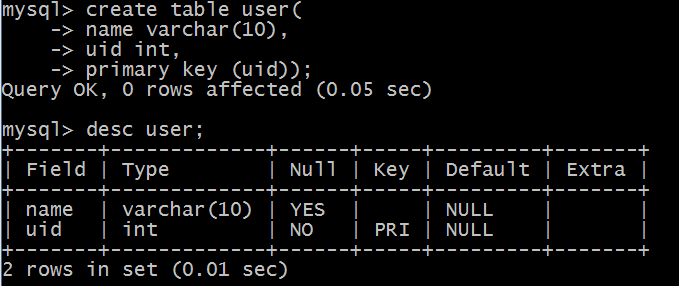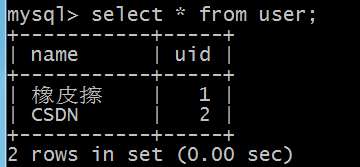## python 数据库编程，这篇是针对 mysql 的，滚雪球学Python第4季第13篇

``    <link rel="stylesheet" href="https://csdnimg.cn/release/blogv2/dist/mdeditor/css/editerView/ck_htmledit_views-1a85854398.css">``

mysql 的安装博客：补充知识：2021 年 mysql 最新 windows 安装教程，滚雪球学 python 第四季。

## mysql 一些简单命令

``````show databases;
``````

``````help
``````

``````exit
``````

``````create database cadb;
``````

``````drop database cadb;
``````

``````use cadb;
``````

``````show tables;
``````

``````desc 表名;
``````

## python 操作 mysql

``````show databases;
-- 建表语句
create table user(name varchar(10),uid int,primary key(uid));

desc user;
````````````insert into user(name,uid) values ("橡皮擦",1);
insert into user(name,uid) values ("CSDN",2);
``````

``````select * from user;
``````1. 连接数据库，生成连接对象；
2. 创建游标对象，用于访问数据表；
3. 执行 sql 语句；
4. 关闭游标；
5. 关闭连接。

python 查询数据
python 连接数据库获取数据

``````import pymysql

# python 连接数据库，返回数据库连接对象
print(conn)
``````

``````<pymysql.connections.Connection object at 0x00000000021E2F60>
``````

``````# 通过 cursor() 创建游标对象，并让查询结果以字典格式输出
cur = conn.cursor(cursor=pymysql.cursors.DictCursor)
# 输出 <pymysql.cursors.DictCursor object at 0x0000000002C03B00>
``````

``````cur.execute("select * from user")
``````

``````# 获取查询结果
data = cur.fetchall()
``````

``````[{'name': '橡皮擦', 'uid': 1}, {'name': 'CSDN', 'uid': 2}]
``````

``````# 关闭游标
cur.close()
# 关闭数据库连接
conn.close()
``````

``````import pymysql

# python 连接数据库，返回数据库连接对象
# print(conn)
with conn.cursor(cursor=pymysql.cursors.DictCursor) as cur:
cur.execute("select * from user where uid = %s", 1)
# 获取查询结果
data = cur.fetchall()
print(data)

# 关闭数据库连接
conn.close()
``````

``````import pymysql

# python 连接数据库，返回数据库连接对象
# print(conn)
with conn.cursor(cursor=pymysql.cursors.DictCursor) as cur:
cur.execute("select * from user where uid = %(uid)s", {"uid": 1})
# 获取查询结果
data = cur.fetchall()
print(data)

# 关闭数据库连接
conn.close()
``````

python 更新数据表数据

``````import pymysql

# python 连接数据库，返回数据库连接对象
# print(conn)
with conn.cursor(cursor=pymysql.cursors.DictCursor) as cur:
try:
cur.execute("update user set name =%s where uid = %s", ["Code", 2])

conn.commit()  # 提交事务
except Exception as e:
print("数据更新异常", e)
conn.rollback()  # 数据回滚

# 关闭数据库连接
conn.close()
``````

`cursor. execute` 可以返回受影响行数，可以直接通过该值判断是否修改成功。

• StandardError
• Warning
• Error
• InterfaceError
• DatabaseError
• DataError
• OperationalError
• IntegrityError
• ProgrammingError
• NotSupportedError

python 新增数据表数据

``````import pymysql

# python 连接数据库，返回数据库连接对象
# print(conn)
with conn.cursor(cursor=pymysql.cursors.DictCursor) as cur:
try:
affected = cur.execute("insert into user(name,uid) values (%s,%s)", ["Good", 3])

conn.commit()  # 提交事务
print("受影响行数", affected)
except Exception as e:
print("数据插入异常", e)
conn.rollback()  # 数据回滚

# 关闭数据库连接
conn.close()
``````

python 删除表格数据

``````affected = cur.execute("delete from user where uid = %s", )
conn.commit()  # 提交事务
print("受影响行数", affected)
``````

## python 操作 nosql 之 dbm

mysql 数据关系型数据库，与之对应的就是非关系型数据库，例如 python 内置的 dbm（database manager ）

``````def open(file, flag='r', mode=0o666):
``````

flag 参数：

• `r` : 只读；
• `w` : 只写；
• `n` : 总是创建一个数据库，打开方式为读写；
• `c` : 存在不创建，不存在则创建。

``````with dbm.open(xxx) as db:
pass
``````

dbm 存储的形式类似 python 字典，以键值对形式存在。

``````import dbm

with dbm.open('example.db', 'n') as db:
db['name'] = '橡皮擦' # 存储值
db['age'] = '18' # values must be bytes or strings
``````

``````import dbm

with dbm.open('example.db', 'r') as db:
ca = db["name"]
age = db["age"]
print(ca)
``````

`dbm` 数据库保存的数据是字符串类型或者字节序列类型，读取到的值是字节序列类型，需要使用解码函数 `decode` 转换成字符串。

## 写在后面

• 滚雪球学 Python（完结）
• 滚雪球学 Python 第二轮（完结）
• 滚雪球学 Python 第三轮
• 滚雪球学 Python 番外篇（完结）
``            <link href="https://csdnimg.cn/release/blogv2/dist/mdeditor/css/editerView/markdown_views-d7a94ec6ab.css" rel="stylesheet">``